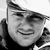# QlikView App Dev

Discussion Board for collaboration related to QlikView App Development.

Announcements
Welcome to Qlik Community! Check out our new navigation! FIND OUT MORE
cancel
Showing results for
Did you mean:Contributor III

## SET ANALYSIS USING VARIABLE AS FIELD IN CONDITION

Hi All,

I wrote the below expression but when using variable as filed in the condition, it does not work.

It seems to be an issue with the syntax of the field (or variable) v(Metric%).

I need it to be a dynamic filed name, as the condition changes with the fields.

Basically i want my expression to divide the count of 'ON TIME' for the selected dynamic field divided by the sum of 'ON TIME' and 'LATE' for the selected dynamic field.

So i need both fields in red and green to be dynamic.

The ones in green seem to work fine, but not the ones in red.

Can you help ?

COUNT(DISTINCT{<[MonthID2]={">=\$(vPrevMonth5ID)<=\$(vCurrMonthID)"},\$(vMetric%) = {'ON TIME'}>}\$(vMetric%))/
(COUNT(DISTINCT{<[MonthID2]={">=\$(vPrevMonth5ID)<=\$(vCurrMonthID)"},\$(vMetric%)={'ON TIME'}>}\$(vMetric%))+
COUNT(DISTINCT{<[MonthID2]={">=\$(vPrevMonth5ID)<=\$(vCurrMonthID)"},\$(vMetric%)={'ON TIME'}>}\$(vMetric%)))

Labels (1)
• ### Set Analysis Syntax Error

5 RepliesPartner - Specialist
Hi Maudifer,
Because I don't have your sample data, but the key to using the variables in set analysis is to keep the data type be same.
In your case, you need to make sure that the data type of MonthID2 is same with your 2 variables:vPrevMonth5ID, vCurrMonthID.
If not, you can use function to change your data type of your 2 variables.
Aiolos ZhaoMVP
Maybe it is the whitespace of you field name that is causing you trouble?

Try:
COUNT(DISTINCT{<[MonthID2]={">=\$(vPrevMonth5ID)<=\$(vCurrMonthID)"},[\$(vMetric%)] = {'ON TIME'}>}[\$(vMetric%)])/
(COUNT(DISTINCT{<[MonthID2]={">=\$(vPrevMonth5ID)<=\$(vCurrMonthID)"},[\$(vMetric%)]={'ON TIME'}>}[\$(vMetric%)])+
COUNT(DISTINCT{<[MonthID2]={">=\$(vPrevMonth5ID)<=\$(vCurrMonthID)"},[\$(vMetric%)]={'ON TIME'}>}[\$(vMetric%)]))Master II

As @Vegar  pointed out the white space might be causing the issue, if not then you can try something similar to below ans check if it works for you

='sum({<'  &  [\$(variable)] & '={1,2,3}>} field)'

Learning never stops.Contributor III
Author

Hi Guys,

thanks you all for your suggestions, but unfortunately nothing worked.

I put below the full correct expression I need. With just the normal field, it works fine, but when i switch the normal field  with a variable, it does not work.I also attached screen shot of the 2 expressions in set analysis, so you can see what gets line in red there. Basically it seems to not like the value condition. You can see below the definition of the variable too.

All the fields's values are either 'ON TIME' OR 'LATE'.

Any other idea on how to fix it ?

Expression with normal field:

COUNT(DISTINCT{<[MonthID2]={'>=\$(vPrevMonth5ID)<=\$(vCurrMonthID)'},[FR VS ATD]={'ON TIME'}>}[Bill of Lading (BOL)])/
(COUNT(DISTINCT{<[MonthID2]={'>=\$(vPrevMonth5ID)<=\$(vCurrMonthID)'},[FR VS ATD]={'ON TIME'}>}[Bill of Lading (BOL)])+
COUNT(DISTINCT{<[MonthID2]={'>=\$(vPrevMonth5ID)<=\$(vCurrMonthID)'},[FR VS ATD]={'LATE'}>}[Bill of Lading (BOL)]))

Expression with Variable field:

COUNT(DISTINCT{<[MonthID2]={'>=\$(vPrevMonth5ID)<=\$(vCurrMonthID)'},\$(vMetric%)={'ON TIME'}>}[Bill of Lading (BOL)])/
(COUNT(DISTINCT{<[MonthID2]={'>=\$(vPrevMonth5ID)<=\$(vCurrMonthID)'},\$(vMetric%)={'ON TIME'}>}[Bill of Lading (BOL)])+
COUNT(DISTINCT{<[MonthID2]={'>=\$(vPrevMonth5ID)<=\$(vCurrMonthID)'},\$(vMetric%)={'LATE'}>}[Bill of Lading (BOL)]))

Variable (\$(vMetric%)) definition:

if(Metric%='FR VS ATD',[FR VS ATD],
if(Metric%='TT PORT TO PORT', [TT PORT TO PORT],

if(Metric%='ATA TO CC', [ATA TO CC],

if(Metric%='CC TO DL', [CC TO DL],

if(Metric%='TT DOOR TO DOOR', [TT PORT TO PORT],

if(Metric%='BD TO DL', [BD TO DL]
))))))Master II
```COUNT(DISTINCT{<[MonthID2]={'>=\$(vPrevMonth5ID)<=\$(vCurrMonthID)'},\$(=vMetric%)={'ON TIME'}>}[Bill of Lading (BOL)])/
(COUNT(DISTINCT{<[MonthID2]={'>=\$(vPrevMonth5ID)<=\$(vCurrMonthID)'},\$(=vMetric%)={'ON TIME'}>}[Bill of Lading (BOL)])+
COUNT(DISTINCT{<[MonthID2]={'>=\$(vPrevMonth5ID)<=\$(vCurrMonthID)'},\$(=vMetric%)={'LATE'}>}[Bill of Lading (BOL)]))```

Try the above . I hpe it works with the variable you have

if that doesn't work then use your expression and change your variable to the below . notice the '='  i used in the starting , don't omit that.

```=if(Metric%='FR VS ATD',[FR VS ATD],
if(Metric%='TT PORT TO PORT', [TT PORT TO PORT],

if(Metric%='ATA TO CC', [ATA TO CC],

if(Metric%='CC TO DL', [CC TO DL],

if(Metric%='TT DOOR TO DOOR', [TT PORT TO PORT],

if(Metric%='BD TO DL', [BD TO DL]
))))))```
Learning never stops.